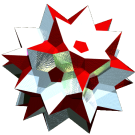Acronym ... Name cubiswirlic heptacontidichoron,twelvefold dissected cubic polytwister,twelvefold dissected cubiter Circumradius sqrt[(3+sqrt(3))/2] = 1.538189 Confer more general: n-cube-swirl   general polytopal classes: isogonal   noble ExternallinksThe Hopf fibration of the cube maps its vertices to according great circles, its edges into twisted (i.e. non-flat but smoothly curved) faces (then looking like a Möbius strip), and the faces get mapped into twisters, which are solid rings bounded by those twisted faces and having thereby throughout the polygonal cross-section of the pre-image, i.e. are squars here. Further each twister then gets dissected into n identical chiral antiprisms. This isochoric construction moreover happens to come out to be isogonal as well, so in total provides a noble polychoron.

This polychoron will have 2 types of edges, one describes the right-up lacing edges of the antiprisms (y), while all its remaining edges belong to the other type (x), simply because the neighbouring twister attaches its cross-secting base polygons next to the left-up lacings of the former. That is, the whole polychoron happens to be chiral in general.

In fact, right this connectedness of the mutually swirling individual twisters does further restrict that n after all. This thus brings back into play the former vertex figure of the starting polyhedron – in addition to the so far only considered faces thereof (the cross-sections of the twisters, i.e. the bases of the antiprisms). Because there also is a full inversion symmetry of the outcome of that fibration, we thus finally have to consideder n = LCM(p, q, 2) for a starting polyhedron {p, q}, i.e. n = LCM(3, 4, 2) = 12 in here.

For that specific value it results in this swirlchoron. Here the edge length ratio can be evaluated as y : x = sqrt[(3-sqrt(3))/2] = 0.796225.

Incidence matrix

96 |  2   6 |   9  3 |  6
---+--------+--------+---
2 | 96   * |   3  0 |  3  y
2 |  * 288 |   2  1 |  3  x
---+--------+--------+---
3 |  1   2 | 288  * |  2
4 |  0   4 |   * 72 |  2
---+--------+--------+---
8 |  4  12 |   8  2 | 72  chiral squap variant